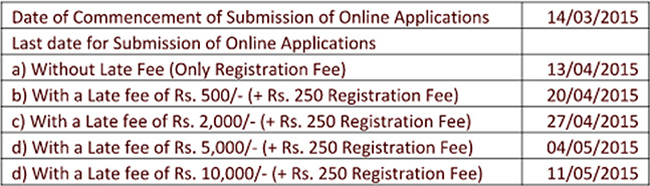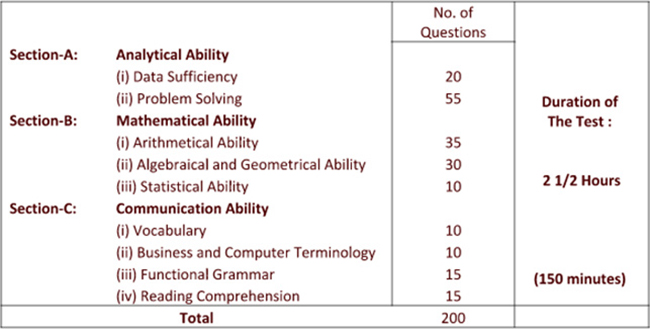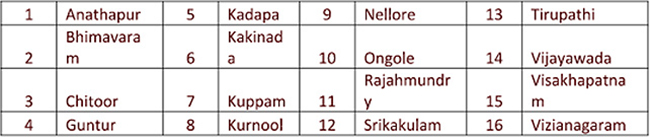# Andhra Pradesh Integrated Common Entrance Test (APICET) – 2015

Andhra Pradesh Integrated Common Entrance Test (APICET-2015) Admission for Master of Computer Application (M.C.A.)

Test Date: 16-05-2015 (Saturday) Timings: 10.00AM to 12.30 P.M.

ONLINE APPLICATION FEE: Rs. 250/- IMPORTANT DATES FOR ONLINE SUBMISSIONSYLLABUS : Section-A: Analytical Ability: 75Q (75 Marks) 1. Data Sufficiency: 20Q (20 Marks) A question is given followed by data in the form of two statements labeled as i and ii. If the data given in i alone is sufficient to answer the question then choice (1) is the correct answer. If the data given in ii alone is sufficient to answer the question then choice (2) is the correct answer. If both i and ii put together are sufficient to answer the question but neither statement alone is sufficient, then choice (3) is the correct answer. If both i and ii put together are not sufficient to answer the question and additional data is needed, then choice (4) is the correct answer. 2. Problem Solving 55Q (55 Marks) a) Sequences and Series 25Q (25 Marks) Analogies of numbers and alphabet, completion of blank spaces following the pattern in a:b::c:d relationship; odd thing out: missing number in a sequence or a series. b) Data Analysis 10Q (10 Marks) The data given in a Table, Graph, Bar diagram, Pie Chart, Venn Diagram or a Passage is to be analyzed and the questions pertaining to the data are to be answered. c) Coding and Decoding Problems 10Q (10 Marks) A code pattern of English Alphabet is given. A given word or a group of letters are to be coded or decoded based on the given code or codes. d) Date, Time & Arrangement Problems 10Q (10 Marks) Calendar problems, clock problems, blood relationships, arrivals, departures and schedules, seating arrangements, symbol and notation interpretation. Section -B: Mathematical Ability 75Q (75 Marks) 1. Arithmetical Ability 35Q (35 Marks) Laws of indices, ratio and proportion; surds; numbers and divisibility, l.c.m. and g.c.d; Rational numbers, Ordering.; Percentages; Profit and loss; Partnership, Pipes and cisterns, time, distance and work problems, areas and volumes, mensuration, modular arithmetic. 2. Algebraical and Geometrical Ability 30Q (30 Marks) Statements, Truth tables, implication converse and inverse, Tautologies-Sets, Relations and functions, applications - Equation of a line in different forms. Trigonometry - Trigonometric ratios, Trigonometric ratios of standard angles, (0°, 30°, 45°, 60°, 90°, 180°): Trigonometric identities: sample problems on heights and distances, Polynomials; Remainder theorem and consequences; Linear equations and expressions; Progressions, Binomial Theorem, Matrices, Notion of a limit and derivative; Plane geometry - lines, Triangles, Quadrilaterals, Circles, Coordinate geometry-distance between points. 3. Statistical Ability: 10Q (10 Marks) Frequency distributions, Mean, Median, Mode, Standard Deviations, Correlation, simple problems on Probability. Section-C: Communication Ability: 50Q (50 Marks) Objectives of the Test Candidates will be assessed on the ability to 1. identify vocabulary used in the day-to-day communication. 2. understand the functional use of grammar in day-to-day communication as well as in the business contexts. 3. identify the basic terminology and concepts in computer and business contexts (letters, reports, memoranda, agenda, minutes etc.). 4. understand written text and drawing inferences. Part 1. Vocabulary 10Q (10M) Part 2. Business and Computer terminology 10Q (10M) Part 3. Functional Grammar 15Q (15M) Part 4. Reading Comprehension (3 Passages) 15Q (15M) GENERAL INFORMATION: The test is designed to measure the candidate's ability to think systematically, to use the verbal and mathematical skills and to assess his/her aptitude for admission into MBA/MCA programme. The Test emphasizes accuracy. Therefore, the candidate is required to go through the instructions carefully. This is an objective type test and the questions are of multiple choice. Out of the given options, the candidate has to choose the correct answer. If the Candidate gives more than one answer to any question, such answers will be ignored while awarding marks. PATTERN OF THE TEST:  The test consists of 200 questions of one mark each in the following topics.REGIONAL TEST CENTERS: The APICET-2015 examination will be held at the following 16 Regional Test Centers in Andhra Pradesh State